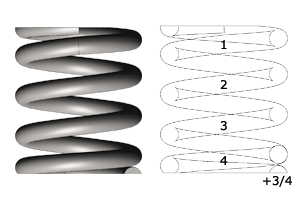# How do you count the number of coils in a compression spring?

The number of coils has a large impact on the function of the compression spring.

The number of coils on the compression spring is defined as:

- n = Number of active coils
- nt = Total number of coils

In the example below, the compression spring has 2.75 n. = 2.75 active coils that absorb the travel and define travel/force.

In the example below the compression spring has 4.75 nt. = 4.75 total coils.